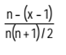# Methods of Depreciation

There are several methods of allocating depreciation over the useful life of the assets. Those employed in industrial and commercial companies use the straightline method and the reducing balance method. The management of a business opts for the most appropriate method(s). A combination of more than one method is sometimes used. In respect of depreciable assets which do not have material value, depreciation is often allocated fully in the accounting period in which they are acquired, e.g. books.

• Straight Line Method: An equal amount is written off every year during the working life on an asset so as to reduce the cost of the asset to NIL or to its residual value at the end of its useful life.

Straight Line Depreciation = (Cost of Asset – Scrap Value)/Useful Life

Depreciation Rate = (Straight Line Depreciation x 100)/Cost of Asset

This method of charging depreciation is recommended mostly for power generating units or for the assets where danger of obselence is low.

• Reducing Balance/Written Down Value Method: A fixed percentage of the diminishing value of the asset is written off each year so as to reduce the asset to its salvage value at the end of its life.

N=useful life

This method is recommended for manufacturing units.

Distinction between Straight Line and Written Down Value Method:

• Sum of the Year Digit Method (SYD): Under this method, the rate of depreciation is charged on the original cost. However, the rate of depreciation for each year is a fraction in which the denominator is the sum of the year digits from 1 to n and numerator for the first year is n, for the second year n-1, for the third year n-2 and so on.

Rate of depreciation for each year is =Where, n = useful life of the asset.

x = number of years asset is in use.

• Machine Hour Method: Where it is practically possible to keep a record of the actual running hours of each machine, depreciation may be calculated on the basis of hours that the concerned machine worked.

Depreciation for year ‘n’ =

This method is recommended for the assets mainly machinery, where in the cost of the asset was determined mainly based on the useful working hours of the machine.

• Depletion Method: This method is used in case of mines, quarries etc. containing only a certain quantity of product. The depreciation rate is calculated by dividing the cost of the asset by the estimated quantity of product likely to be available.

Depreciation for year ‘n’ =

Annuity Method: This is a method of depreciation which also takes into account the element of interest on capital outlay and seeks to write off the value of the asset as well as the interest lost over the life of the asset. On that basis, the amount of depreciation to be annually provided in the account is ascertained from the Annuity Tables. Though the amount written off annually is constant, the interest in the earlier years being greater, only small amount of the capital outlay is written off. This proportion is reversed with the passage of time.

This method of charging depreciation is mostly recommended for leasehold assets.

• Sinking Fund Method: If the sum involved in replacing the asset is large, than just providing for depreciation will not be sufficient, as because the concern may not have the ready fund available to replace the assets. For this purpose a Sinking Fund Account is created, a depreciation amount is credited to it. The amount is invested in some Government Securities. Every year the process is repeated, the interest received from such securities is also reinvested and these securities are sold at the end of the life of the asset, so that the concern has the ready fund to replace the asset.

Disposal of Assets

Where depreciable assets are disposed of, discarded, demolished or destroyed, the net surplus or deficiency, if material, is disclosed separately.